https://doi.org/10.5194/gmd-12-1009-2019
https://doi.org/10.5194/gmd-12-1009-2019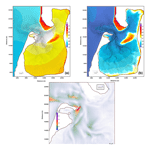# FESOM-C v.2: coastal dynamics on hybrid unstructured meshes

Alexey Androsov, Vera Fofonova, Ivan Kuznetsov, Sergey Danilov, Natalja Rakowsky, Sven Harig, Holger Brix, and Karen Helen Wiltshire
Abstract

We describe FESOM-C, the coastal branch of the Finite-volumE Sea ice – Ocean Model (FESOM2), which shares with FESOM2 many numerical aspects, in particular its finite-volume cell-vertex discretization. Its dynamical core differs in the implementation of time stepping, the use of a terrain-following vertical coordinate, and the formulation for hybrid meshes composed of triangles and quads. The first two distinctions were critical for coding FESOM-C as an independent branch. The hybrid mesh capability improves numerical efficiency, since quadrilateral cells have fewer edges than triangular cells. They do not suffer from spurious inertial modes of the triangular cell-vertex discretization and need less dissipation. The hybrid mesh capability allows one to use quasi-quadrilateral unstructured meshes, with triangular cells included only to join quadrilateral patches of different resolution or instead of strongly deformed quadrilateral cells. The description of the model numerical part is complemented by test cases illustrating the model performance.

Share
Dates
1 Introduction

Many practical problems in oceanography require regional focus on coastal dynamics. Although global ocean circulation models formulated on unstructured meshes may in principle provide local refinement, such models are as a rule based on assumptions that are not necessarily valid in coastal areas. The limitations on dynamics coming from the need to resolve thin layers, maintain stability for sea surface elevations comparable to water layer thickness, or simulate the processes of wetting and drying make the numerical approaches traditionally used in coastal models different from those used in large-scale models. For this reason, combining coastal and large-scale functionality in a single unstructured-mesh model, although possible, would still imply a combination of different algorithms and physical parameterizations. Furthermore, on unstructured meshes, the numerical stability of open boundaries, needed in regional configurations, sometimes requires masking certain terms in motion equations close to open boundaries. This would be an unnecessary complication for a large-scale unstructured-mesh model that is as a rule global.

The main goal of the development described in this paper was to design a tool, dubbed FESOM-C, that is close to FESOM2 in its basic principles, but can be used as a coastal model. Its routines handling the mesh infrastructure are derived from FESOM2. However, the time stepping, vertical discretization, and particular algorithms, detailed below, are different. FESOM-C relies on a terrain-following vertical coordinate (vs. the arbitrary Lagrangian–Eulerian (ALE) vertical coordinate of FESOM2), but takes a step further with respect to the mesh structure. It is designed to work on hybrid meshes composed of triangles and quads. Some decisions, such as the lack of the ALE at the present stage, are only motivated by the desire to keep the code as simple as possible through the initial phase of its development and maintenance. The code is based on the cell-vertex finite-volume discretization, the same as FESOM2 and FVCOM . It places scalar quantities at mesh vertices and the horizontal velocities at cell centroids.

Our special focus is on using hybrid meshes. In essence, the capability of hybrid meshes is built on the finite-volume method. Indeed, computations of fluxes are commonly implemented as cycles over edges, and the edge-based infrastructure is immune to the polygonal type of mesh cells. However, because of staggering, it is still convenient to keep some computations on cells, which then depend on the cell type. Furthermore, high-order transport algorithms might also be sensitive to the cell geometry. We limit the allowed polygons to triangles and quads. Although there is no principal limitation on the polygon type, triangles and quads are versatile enough in practice for the cell-vertex discretization. Our motivation for using quads is twofold . First, quadrilateral meshes have 1.5 times fewer edges than triangular meshes, which speeds up computations because cycles over edges become shorter. The second reason is the intrinsic problem of the triangular cell-vertex discretization – the presence of spurious inertial modes (see, e.g., ) and decoupling between the nearest horizontal velocities. Although both can be controlled by lateral viscosity, the control leads to higher viscous dissipation over the triangular portions of the mesh. The hybrid meshes can be designed so that triangular cells are included only to optimally match the resolution or are even absent altogether. For example, FESOM-C can be run on curvilinear meshes combining smooth changes in the shape of quadrilateral cells with smoothly approximated coastlines. One can also think of meshes for which triangular patches are only used to provide transitions between quadrilateral parts of different resolution, implementing an effective nesting approach.

Many unstructured-mesh coastal ocean models were proposed recently . It will take some time for FESOM-C to catch up in terms of functionality. The decision on the development of FESOM-C was largely motivated by the desire to fit in the existing modeling infrastructure (mesh design, analysis tools, input–output organization), and not by any deficiency in existing models. The real workload was substantially reduced through the use or modification of the existing FESOM2 routines.

We formulate the main equations and their discretization in the three following sections. Section 5 presents results of test simulations, followed by a discussion and conclusions.

2 Model formulation

## 2.1 The governing equations

We solve standard primitive equations in the Boussinesq, hydrostatic, and traditional approximations (see, e.g., ). The solution is sought in the domain $\stackrel{\mathrm{^}}{Q}=Q×\left[\mathrm{0},{t}_{\mathrm{f}}\right]$, where tf is the time interval. The boundary Q of domain Q is formed by the free water surface, the bottom boundaries, and lateral boundaries composed of the solid part Q1 and the open boundary Q2, $Q=\mathit{\left\{}x,y,z;x,y\in \mathrm{\Omega },-h\left(x,y\right)\le z<\mathit{\zeta }\left(x,y,t\right)\mathit{\right\}}$, $\mathrm{0}\le t\le {t}_{\mathrm{f}}$. Here ζ is the surface elevation and h the bottom topography. We seek the vector of unknown $\mathbit{q}=\left(\mathbit{u},w,\mathit{\zeta },T,S\right)$, where $\mathbit{u}=\left(u,v\right)$ is the horizontal velocity, w the vertical velocity, T the potential temperature, and S the salinity, satisfying the equations

$\begin{array}{ll}& \frac{\partial \mathbit{u}}{\partial t}+\frac{\partial }{\partial {x}_{i}}\left(\mathbit{u}{u}_{i}\right)+\frac{\partial }{\partial z}\left(\mathbit{u}w\right)+\frac{\mathrm{1}}{{\mathit{\rho }}_{\mathrm{0}}}\mathrm{\nabla }p+f\mathbit{k}×\mathbit{u}\\ \text{(1)}& & \phantom{\rule{0.25em}{0ex}}\phantom{\rule{0.25em}{0ex}}\phantom{\rule{0.25em}{0ex}}\phantom{\rule{0.25em}{0ex}}=\frac{\partial }{\partial z}\mathit{\vartheta }\frac{\partial \mathbit{u}}{\partial z}+\mathrm{\nabla }\cdot \left(K\mathrm{\nabla }\right)\mathbit{u},\text{(2)}& & \mathrm{\nabla }\cdot \mathbit{u}+\frac{\partial }{\partial z}w=\mathrm{0},\text{(3)}& & \frac{\partial p}{\partial z}=-g\mathit{\rho },& \frac{\partial {\mathrm{\Theta }}_{j}}{\partial t}+\frac{\partial }{\partial {x}_{i}}\left({\mathbit{u}}_{i}{\mathrm{\Theta }}_{j}\right)+\frac{\partial }{\partial z}\left(w{\mathrm{\Theta }}_{j}\right)=\frac{\partial }{\partial z}{\mathit{\vartheta }}_{\mathrm{\Theta }}\frac{\partial {\mathrm{\Theta }}_{j}}{\partial z}\\ \text{(4)}& & \phantom{\rule{0.25em}{0ex}}\phantom{\rule{0.25em}{0ex}}\phantom{\rule{0.25em}{0ex}}\phantom{\rule{0.25em}{0ex}}+\mathrm{\nabla }\left({K}_{\mathrm{\Theta }}\mathrm{\nabla }\right){\mathrm{\Theta }}_{j}.\end{array}$

Here $i=\mathrm{1},\mathrm{2}$, x1=x, x2=y, u1=u, and u2=v, and summation is implied over the repeating indices i; p is the pressure; and $j=\mathrm{1},\mathrm{2}$ with Θ1=T and Θ2=S represents the potential temperature and salinity, respectively. The seawater density is determined by the equation of state $\mathit{\rho }=\mathit{\rho }\left(T,S,p\right)$, and ρ0 is the reference density; f is the Coriolis parameter; k is the vertical unit vector; ϑ and K are the coefficients of vertical and horizontal turbulent momentum exchange, respectively; ϑΘ and KΘ are the respective diffusion coefficients; and g is the acceleration due to gravity.

Writing

$\begin{array}{}\text{(5)}& \mathit{\rho }\left(x,y,z,t\right)={\mathit{\rho }}_{\mathrm{0}}+{\mathit{\rho }}^{\prime }\left(x,y,z,t\right),\end{array}$

where ρ is the density fluctuation, we obtain, integrating Eq. (3),

$p-{p}_{\mathrm{atm}}=\underset{z}{\overset{\mathit{\zeta }}{\int }}\mathit{\rho }g\mathrm{d}z=g{\mathit{\rho }}_{\mathrm{0}}\left(\mathit{\zeta }-z\right)+g\underset{z}{\overset{\mathit{\zeta }}{\int }}{\mathit{\rho }}^{\prime }\mathrm{d}z,$

where patm is the atmospheric pressure. The horizontal pressure gradient is then expressed as the sum of barotropic, baroclinic, and atmospheric pressure gradients:

$\begin{array}{ll}& {\mathit{\rho }}_{\mathrm{0}}^{-\mathrm{1}}\mathrm{\nabla }p=g\mathrm{\nabla }\mathit{\zeta }+g{\mathit{\rho }}_{\mathrm{0}}^{-\mathrm{1}}\mathrm{\nabla }I+{\mathit{\rho }}_{\mathrm{0}}^{-\mathrm{1}}\mathrm{\nabla }{p}_{\mathrm{atm}},\\ \text{(6)}& & I=\underset{z}{\overset{\mathit{\zeta }}{\int }}{\mathit{\rho }}^{\prime }\mathrm{d}z.\end{array}$

Note that horizontal derivatives here are taken at fixed z.

## 2.2 Turbulent closures

The default scheme to compute the vertical viscosity and diffusivity in the system of Eqs. (1)–(4) is based on the Prandtl–Kolmogorov hypothesis of incomplete similarity. According to it, the turbulent kinetic energy b, the coefficient of turbulent mixing ϑ, and dissipation of turbulent energy ε are connected as $\mathit{\vartheta }=l\sqrt{b}$, where l is the scale of turbulence, ϑΘ=cρϑ, $\mathit{\epsilon }={c}_{\mathit{\epsilon }}{b}^{\mathrm{2}}/\mathit{\vartheta }$, and cε=0.046 . Prandtl's number cρ is commonly chosen as 0.1 and sets the relationship between the coefficients of turbulent diffusion and viscosity. The equation describing the balance of turbulent kinetic energy is obtained by parameterizing the energy production and dissipation in the equation for turbulent kinetic energy b as

$\begin{array}{}\text{(7)}& \frac{\partial b}{\partial t}-\mathit{\vartheta }\left({\left|{\mathbit{u}}_{z}\right|}^{\mathrm{2}}+{c}_{\mathit{\rho }}g{\mathit{\rho }}_{\mathrm{0}}^{-\mathrm{1}}\frac{\partial \mathit{\rho }}{\partial z}\right)+{c}_{\mathit{\epsilon }}{b}^{\mathrm{2}}/\mathit{\vartheta }={\mathit{\alpha }}_{b}\frac{\partial }{\partial z}\mathit{\vartheta }\frac{\partial b}{\partial z},\end{array}$

with the boundary conditions

$\begin{array}{ll}& b{|}_{h}={B}_{\mathrm{1}}|\mathbit{u}{|}^{\mathrm{2}},\\ & \mathit{\vartheta }{b}_{z}{|}_{\mathit{\zeta }}={\mathit{\gamma }}_{\mathit{\zeta }}{u}_{*\mathit{\zeta }}^{\mathrm{3}},\end{array}$

where αb=0.73, B1=16.6, and ${\mathit{\gamma }}_{\mathit{\zeta }}=\mathrm{0.4}×{\mathrm{10}}^{-\mathrm{3}}$; ${u}_{*\mathit{\zeta }}=\left(\mathit{\rho }/{\mathit{\rho }}_{\mathrm{a}}{\right)}^{\mathrm{1}/\mathrm{2}}{u}_{*}$ is the dynamical velocity in water near the surface, ρa the air density, and u* the dynamic velocity of water on the interface between air and water.

Dissipative term is written as

${b}^{\mathrm{2}}/\mathit{\vartheta }=\left(\mathrm{2}{b}^{\mathit{\nu }+\mathrm{1}}{b}^{\mathit{\nu }}-\left({b}^{\mathrm{2}}{\right)}^{\mathit{\nu }}\right)/{\mathit{\vartheta }}^{\mathit{\nu }},$

where ν is the index of iterations. Equation (7) is solved by a three-point Thomas scheme in the vertical direction with the boundary conditions given above. Iterations are carried out until convergence determined by the condition

$max\left|\left({b}^{\mathit{\nu }+\mathrm{1}}-{b}^{\mathit{\nu }}\right)/{b}^{\mathit{\nu }}\right|<\mathit{\varpi },$

where ϖ is a small value O(10−6). More details on the solution of this equation are given in .

To determine the turbulence scale l in the presence of surface and bottom boundary layers we use the Montgomery formula (Reid1957):

$l=\frac{\mathit{\kappa }}{H}{Z}_{h}{Z}_{\mathit{\zeta }},$

where $H=h+\mathit{\zeta }$ is the full water depth, ${Z}_{h}=z+h+{z}_{h}$, ${Z}_{\mathit{\zeta }}=-z+\mathit{\zeta }+{z}_{\mathit{\zeta }}$, κ≃0.4 is the von Kármán constant, z the layer depth, and zh and zζ are the roughness parameters for the bottom and free surface, respectively. To remove turbulent mixing in layers that are distant from interfaces we modify the Montgomery formula by introducing the cutoff function ${Z}_{\mathrm{0}}=\mathrm{1}-{\mathit{\beta }}_{\mathrm{1}}{H}^{-\mathrm{2}}{Z}_{h}{Z}_{\mathit{\zeta }}$, $\mathrm{0}\le {\mathit{\beta }}_{\mathrm{1}}\le \mathrm{4}$ :

$l=\frac{\mathit{\kappa }}{H}{Z}_{h}{Z}_{\mathit{\zeta }}{Z}_{\mathrm{0}}.$

In addition to the default scheme, one may select a scheme provided by the General Ocean Turbulence Model (GOTM) implemented into the FESOM-C code for computing vertical eddy viscosity and diffusion for momentum and tracer equations. GOTM includes large number of well-tested turbulence models with at least one member of every relevant model family (empirical models, energy models, two-equation models, algebraic stress models, K-profile parameterizations, etc.) and treats every single water column independently. An essential part of GOTM includes one-point second-order schemes .

## 2.3 Bottom friction parameterization

The model uses either a constant bottom friction coefficient Cd, or it is computed through the specified bottom roughness height zh. The first option is preferable if the vertical resolution everywhere in the domain does not resolve the logarithmic layer or when the vertically averaged equations are solved. In the second option the bottom friction coefficient is computed according to and has the following form:

${C}_{\mathrm{d}}={\left(\mathrm{ln}\left(\left(\mathrm{0.5}{h}_{\mathrm{b}}+{z}_{h}\right)/{z}_{h}\right)/\mathit{\kappa }\right)}^{-\mathrm{2}},$

where hb is the thickness of the bottom layer. It is also possible to prescribe Cd or zh as a function of the horizontal coordinate at the initialization step.

## 2.4 Boundary conditions

The boundary conditions for the dynamical Eqs. (1)–(2) are those of no-slip on the solid boundary Q1,

As is well known, the formulation of open boundary conditions faces difficulties. They are related to either the lack or incompleteness of information demanded by the theory, for example on velocity components at the open boundary. Furthermore, whatever the external information, it may contradict the solution inside the computational domain, leading to instabilities that are frequently expressed as small-scale vortex structures forming near the open boundary. The procedure reconciling the external information with the solution inside the domain becomes of paramount importance. We use two approaches. The first one is to use a function whereby advection and horizontal diffusion are smoothly tapered to zero in the close vicinity of open boundary Q2. Such tapering makes the equations quasi-hyperbolic at the open boundary so that the formulation of one condition (for example, for the elevation, ) is possible .

The other approach is to adapt the external information. It is applied to scalar fields and will be explained further.

Note that despite simplifications, barotropic and baroclinic perturbations may still disagree at the open boundary, leading to instabilities in its vicinity. In this case an additional buffer zone is introduced with locally increased horizontal diffusion and bottom friction.

Dynamic boundary conditions on the top and bottom specify the momentum fluxes entering the ocean. Neglecting the contributions from horizontal viscosities, we write

The first one sets the surface momentum flux to the wind stress at the surface (τζ), and the second one sets the bottom momentum flux to the frictional flux at the bottom (τh), with uh the bottom velocity.

Now we turn to the boundary conditions for the scalar quantities obeying Eq. (4). This is a three-dimensional parabolic equation and the boundary conditions are determined by its leading (diffusive) terms. We impose the no-flux condition on the solid boundary Q1 and the bottom $z=-h$.

The conditions at the open boundary Q2 are given for outflow and inflow; see, e.g., and :

${\mathrm{\Theta }}_{t}+a{\mathrm{\Theta }}_{x}+b{\mathrm{\Theta }}_{y}=-\frac{\mathrm{1}}{\mathit{\tau }}\left(\mathrm{\Theta }-{\mathrm{\Theta }}_{\mathrm{\Gamma }}\right),$

where ΘΓ is the given field value, usually a climatological one or relying on data from a global numerical model or observations. If the phase velocity components and $a=-{\mathrm{\Theta }}_{t}{\mathrm{\Theta }}_{x}/G$, $b=-{\mathrm{\Theta }}_{t}{\mathrm{\Theta }}_{y}/G$, and $G=\left[\left(\partial \mathrm{\Theta }/\partial x{\right)}^{\mathrm{2}}+\left(\partial \mathrm{\Theta }/\partial y{\right)}^{\mathrm{2}}{\right]}^{-\mathrm{1}}$ , Θ propagates out of the domain, and one sets τ=τ0. If it propagates into the domain, a and b are set to zero and τ=τΓ, with τΓτ0. The parameter τ is determined experimentally and commonly is from hours to days. In the FESOM-C such an adaptive boundary condition is routinely applied for temperature and salinity, yet it can also be used for any components of a solution.

At the surface the fluxes are due to the interaction with the atmosphere:

where $\stackrel{\mathrm{^}}{Q}$ is the heat flux excluding shortwave radiation, which has been included as a volume heat source in the temperature equation, with cp the specific heat of seawater. The impact of the precipitation–evaporation has been included as a volume source in the continuity equation. In the presence of rivers, their discharge is added either as a prescribed inflow at the open boundary in the river mouth or as volume sources of mass, heat, and momentum distributed in the vicinity of the open boundary. In the first case it might create an initial shock in elevation, so the second method is safer.

3 Temporal discretization

As is common in coastal models, we split the fast and slow motions into, respectively, barotropic and baroclinic subsystems . The reason for this splitting is that surface gravity waves (external mode) are fast and impose severe limitations on the time step, whereas the internal dynamics can be computed with a much larger time step. The time step for the external mode τ2-D is limited by the speed of surface gravity waves, and that for the internal mode, τ3-D, by the speed of internal waves or advection. The ratio ${M}_{t}={\mathit{\tau }}_{\mathrm{3}\text{-}\mathrm{D}}/{\mathit{\tau }}_{\mathrm{2}\text{-}\mathrm{D}}$ depends on applications, but is commonly between 10 and 30. In practice, additional limitations are due to vertical advection or wetting and drying processes. We will further use the indices k and n to enumerate the internal and external time steps, respectively.

The numerical algorithm passes through several stages. In the first stage, based on the current temperature and salinity fields (time step k), the pressure is computed from hydrostatic equilibrium Eq. (6) and then used to compute the baroclinic pressure gradient ${\mathit{\rho }}_{\mathrm{0}}^{-\mathrm{1}}\mathrm{\nabla }p=g\mathrm{\nabla }{\mathit{\zeta }}^{k}+g{\mathit{\rho }}_{\mathrm{0}}^{-\mathrm{1}}\mathrm{\nabla }{I}^{k}+{\mathit{\rho }}_{\mathrm{0}}^{-\mathrm{1}}\mathrm{\nabla }{p}_{\mathrm{atm}}$. We use an asynchronous time stepping, assuming that integration of temperature and salinity is half-step shifted with respect to momentum. The index k on I implies that it is centered between k and k+1 of momentum integration. The elevation in the expression above is taken at time step k, which makes the entire estimate for p only first-order accurate with respect to time.

At the second stage, the predictor values of the three-dimensional horizontal velocity are determined as

$\begin{array}{ll}& {\stackrel{\mathrm{̃}}{\mathbit{u}}}^{k+\mathrm{1}}-{\mathbit{u}}^{k}={\mathit{\tau }}_{\mathrm{3}\text{-}\mathrm{D}}{\left(-f\mathbit{k}×\mathbit{u}-\mathrm{\nabla }\cdot \mathbit{u}\mathbit{u}+\mathrm{\nabla }\cdot \left(K\mathrm{\nabla }\mathbit{u}\right)\right)}^{\mathrm{AB}\mathrm{3}}\\ & \phantom{\rule{0.25em}{0ex}}\phantom{\rule{0.25em}{0ex}}\phantom{\rule{0.25em}{0ex}}\phantom{\rule{0.25em}{0ex}}-{\mathit{\tau }}_{\mathrm{3}\text{-}\mathrm{D}}{\mathit{\rho }}_{\mathrm{0}}^{-\mathrm{1}}\mathrm{\nabla }p+{\mathit{\tau }}_{\mathrm{3}\text{-}\mathrm{D}}{\partial }_{z}\mathit{\vartheta }{\partial }_{z}{\stackrel{\mathrm{̃}}{\mathbit{u}}}^{k+\mathrm{1}}-{\mathit{\tau }}_{\mathrm{3}\text{-}\mathrm{D}}{\partial }_{z}{\left(w\mathbit{u}\right)}^{\mathrm{AB}\mathrm{3}}.\end{array}$

Here K is the coefficient of horizontal viscosity, and AB3 implies the third-order Adams–Bashforth estimate. The horizontal viscosity operator can be made biharmonic or replaced with filtering as discussed in the next chapter.

To carry out mode splitting, we write the horizontal velocity as the sum of the vertically averaged one $\stackrel{\mathrm{‾}}{\mathbit{u}}$ and the deviation thereof (pulsation) u:

$\begin{array}{ll}& \mathbit{u}=\stackrel{\mathrm{‾}}{\mathbit{u}}+{\mathbit{u}}^{\prime },\\ & \stackrel{\mathrm{‾}}{\mathbit{u}}=\frac{\mathrm{1}}{H}\underset{-h}{\overset{\mathit{\zeta }}{\int }}\mathbit{u}\mathrm{d}z,\\ & \underset{-h}{\overset{\mathit{\zeta }}{\int }}{\mathbit{u}}^{\prime }=\mathrm{0}.\end{array}$

By integrating the system in Eqs. (1)–(3) vertically between the bottom and surface, with regard for the kinematic boundary conditions ${\partial }_{t}\mathit{\zeta }+\mathbit{u}\mathrm{\nabla }\mathit{\zeta }=w$ on the surface, $-\mathbit{u}\mathrm{\nabla }h=w$ at the bottom, and time discretization, we get

$\begin{array}{ll}& \left({\mathit{\zeta }}^{n+\mathrm{1}}-{\mathit{\zeta }}^{n}\right)+{\mathit{\tau }}_{\mathrm{2}\text{-}\mathrm{D}}\mathrm{\nabla }{\left(H\stackrel{\mathrm{‾}}{\mathbit{u}}\right)}^{\mathrm{AB}\mathrm{3}}=\mathrm{0},\\ & {\stackrel{\mathrm{‾}}{\mathbit{u}}}^{n+\mathrm{1}}-{\stackrel{\mathrm{‾}}{\mathbit{u}}}^{n}={\mathit{\tau }}_{\mathrm{2}\text{-}\mathrm{D}}{\left(-f\mathbit{k}×\stackrel{\mathrm{‾}}{\mathbit{u}}-\mathrm{\nabla }\cdot \stackrel{\mathrm{‾}}{\mathbit{u}}\phantom{\rule{0.125em}{0ex}}\stackrel{\mathrm{‾}}{\mathbit{u}}+\mathrm{\nabla }\cdot \left(K\mathrm{\nabla }\stackrel{\mathrm{‾}}{\mathbit{u}}\right)\right)}^{\mathrm{AB}\mathrm{3}}\\ & \phantom{\rule{0.25em}{0ex}}\phantom{\rule{0.25em}{0ex}}\phantom{\rule{0.25em}{0ex}}\phantom{\rule{0.25em}{0ex}}-{\mathit{\tau }}_{\mathrm{2}\text{-}\mathrm{D}}\left(g\mathrm{\nabla }\mathit{\zeta }{\right)}^{\mathrm{AM}\mathrm{4}}+{\mathit{\tau }}_{\mathrm{2}\text{-}\mathrm{D}}\left({\mathit{\tau }}_{\mathit{\zeta }}/{\mathit{\rho }}_{\mathrm{0}}-{\mathit{\tau }}_{h}\right)-{\mathit{\tau }}_{\mathrm{2}\text{-}\mathrm{D}}{R}_{\mathrm{3}\text{-}\mathrm{D}}^{\prime }\\ & \phantom{\rule{0.25em}{0ex}}\phantom{\rule{0.25em}{0ex}}\phantom{\rule{0.25em}{0ex}}\phantom{\rule{0.25em}{0ex}}-{\mathit{\tau }}_{\mathrm{2}\text{-}\mathrm{D}}g{\mathit{\rho }}_{\mathrm{0}}^{-\mathrm{1}}\mathrm{\nabla }{\stackrel{\mathrm{‾}}{I}}^{k}.\end{array}$

Here a specific version of AB3 is used: ${\stackrel{\mathrm{‾}}{\mathbit{u}}}^{\mathrm{AB}\mathrm{3}}=\left(\mathrm{3}/\mathrm{2}+\mathit{\beta }\right){\stackrel{\mathrm{‾}}{\mathbit{u}}}^{k}-\left(\mathrm{1}/\mathrm{2}+\mathrm{2}\mathit{\beta }\right){\stackrel{\mathrm{‾}}{\mathbit{u}}}^{k-\mathrm{1}}+\mathit{\beta }{\stackrel{\mathrm{‾}}{\mathbit{u}}}^{k-\mathrm{2}}$, with β=0.281105 for stability reasons ; AM4 implies the Adams–Multon estimate ${\mathit{\zeta }}^{\mathrm{AM}\mathrm{4}}=\mathit{\delta }{\mathit{\zeta }}^{n+\mathrm{1}}+\left(\mathrm{1}-\mathit{\delta }-\mathit{\gamma }-\mathit{ϵ}\right){\mathit{\zeta }}^{n}+\mathit{\gamma }{\mathit{\zeta }}^{n-\mathrm{1}}+\mathit{ϵ}{\mathit{\zeta }}^{n-\mathrm{2}}$, taken with δ=0.614, γ=0.088, and ϵ=0.013 . In the equations above τζ and τh are the surface (wind) and bottom stresses, respectively, and $\mathrm{\nabla }\stackrel{\mathrm{‾}}{I}$ is the vertically integrated gradient of baroclinic pressure. The term ${R}_{\mathrm{3}\text{-}\mathrm{D}}^{\prime }$ contains momentum advection and horizontal dissipation of the pulsation velocity integrated vertically:

${R}_{\mathrm{3}\text{-}\mathrm{D}}^{\prime }=\frac{\mathrm{1}}{{H}^{k}}{\left[\underset{-h}{\overset{\mathit{\zeta }}{\int }}\mathrm{\nabla }\cdot {\mathbit{u}}^{\mathbf{\prime }}{\mathbit{u}}^{\mathbf{\prime }}-\underset{-h}{\overset{\mathit{\zeta }}{\int }}\mathrm{\nabla }\cdot \left(K\mathrm{\nabla }{\mathbit{u}}^{\mathbf{\prime }}\right)\right]}^{k}.$

In this expression Hk is the total fluid depth at time step k, ${H}^{k}=h+{\mathit{\zeta }}^{k}$. This term is computed only on the baroclinic time step and kept constant through the integration of the internal mode.

The bottom friction is taken as

${\mathit{\tau }}_{h}={C}_{\mathrm{d}}{\left|\stackrel{\mathrm{‾}}{\mathbit{u}}\right|}^{n}\left({\stackrel{\mathrm{‾}}{\mathbit{u}}}^{n+\mathrm{1}}-{\stackrel{\mathrm{‾}}{\mathbit{u}}}^{n}\right)/{H}^{n+\mathrm{1}}+{C}_{\mathrm{d}}|{\stackrel{\mathrm{̃}}{\mathbit{u}}}_{h}{|}^{k+\mathrm{1}}{\stackrel{\mathrm{̃}}{\mathbit{u}}}_{h}^{k+\mathrm{1}}/{H}^{n+\mathrm{1}}.$

The first part of bottom friction is needed to increase stability, while the second part estimates the correct friction, with ${\stackrel{\mathrm{̃}}{\mathbit{u}}}_{h}^{k+\mathrm{1}}$ the horizontal velocity vector in the bottom cell on the predictor time step.

The system of vertically averaged equations is stepped explicitly (except for the bottom friction) through Mt time steps of duration τ2-D (index n) to “catch up” the k+1 baroclinic time step. The update of elevation is made first, followed by the update of vertically integrated momentum equations.

At the “corrector” step, the 3-D velocities are corrected to the surface elevation at k+1:

${\mathbit{u}}^{k+\mathrm{1}}=\frac{{\mathrm{△}}_{i}^{k}}{{\mathrm{△}}_{i}^{k+\mathrm{1}}}{\stackrel{\mathrm{̃}}{\mathbit{u}}}^{k+\mathrm{1}}+\left({\stackrel{\mathrm{‾}}{\mathbit{u}}}^{k+\mathrm{1}}-{\stackrel{\mathrm{‾}}{\mathbit{u}}}^{P}\right),$

with ${\stackrel{\mathrm{‾}}{\mathbit{u}}}^{P}=\frac{\mathrm{1}}{{H}^{k+\mathrm{1}}}{\sum }_{-h}^{\mathit{\zeta }}\left({\stackrel{\mathrm{̃}}{\mathbit{u}}}^{k+\mathrm{1}}{\mathrm{△}}_{i}^{k}\right)$, where i is the vertical index. Here ${\mathrm{△}}_{i}^{k}$ and ${\mathrm{△}}_{i}^{k+\mathrm{1}}$ are the thicknesses of the ith layer calculated on respective baroclinic time steps. The layer thickness is ${\mathrm{△}}_{i}^{k}={\mathrm{△}}_{i}{H}^{k}$, where i is the unperturbed vertical grid spacing. This correction removes the barotropic component of the predicted velocity and combines the result with the computed barotropic velocity. We will suppress the layer index i where it is unambiguous.

The final step in the dynamical part calculates the transformed vertical velocity wk+1 from 3-D continuity Eq. (2). It is used in the next predictor step. Note that in the predictor step the computations of vertical viscosity are implicit.

New horizontal velocities, the so-called “filtered” ones, are used for advection of a tracer. They are given by the sum of the filtered depth mean and the baroclinic part of the “predicted” velocities ,

${\mathbit{u}}_{F}^{k+\mathrm{1}}=\frac{{\mathrm{△}}_{i}^{k}}{{\mathrm{△}}_{i}^{k+\mathrm{1}}}{\stackrel{\mathrm{̃}}{\mathbit{u}}}^{k+\mathrm{1}}+\left({\stackrel{\mathrm{‾}}{\mathbit{u}}}^{F}-{\stackrel{\mathrm{‾}}{\mathbit{u}}}^{P}\right),$

with ${\stackrel{\mathrm{‾}}{\mathbit{u}}}^{F}=\frac{\mathrm{1}}{{M}_{t}{H}^{k+\mathrm{1}}}{\sum }_{n=\mathrm{1}}^{n={M}_{t}}\left({\stackrel{\mathrm{‾}}{\mathbit{u}}}^{n}{H}^{n}\right)$. The procedure of “filtering” removes possible high-frequency components in the barotropic velocity. It also improves accuracy because it in essence works toward centering the contribution of the elevation gradient. Once the filtered velocity is computed, the vertical velocity is updated to match it.

The equation for temperature is taken in the conservation form

$\begin{array}{ll}& {\mathrm{△}}^{k+\mathrm{1}}{T}^{k+\mathrm{1}}\\ & \phantom{\rule{0.25em}{0ex}}\phantom{\rule{0.25em}{0ex}}\phantom{\rule{0.25em}{0ex}}\phantom{\rule{0.25em}{0ex}}={\mathrm{△}}^{k}{T}^{k}-{\mathit{\tau }}_{\mathrm{3}\text{-}\mathrm{D}}\left[\mathrm{\nabla }\cdot \left({\mathbit{u}}_{F}^{k+\mathrm{1}}{\mathrm{△}}^{k}{T}^{*}\right)+{w}_{\mathrm{Ft}}{T}_{\mathrm{t}}^{*}-{w}_{\mathrm{Fb}}{T}_{\mathrm{b}}^{*}\right]\\ & \phantom{\rule{0.25em}{0ex}}\phantom{\rule{0.25em}{0ex}}\phantom{\rule{0.25em}{0ex}}\phantom{\rule{0.25em}{0ex}}+D+{\mathit{\tau }}_{\mathrm{3}\text{-}\mathrm{D}}R+{\mathit{\tau }}_{\mathrm{3}\text{-}\mathrm{D}}C,\end{array}$

where D combines the terms related to diffusion, wFt,wFb, Tt, and Tb are the vertical transport velocity and temperature on top and bottom of the layer, and T* is computed through the second-order Adams–Bashforth estimate. R is the boundary thermal flux (either from the surface or due to river discharge). The last term in the equation above is

$C=T\frac{\partial {\mathrm{△}}_{i}}{\partial t}=-T\left(\mathrm{\nabla }{\left({\mathrm{△}}_{i}{\mathbit{u}}_{F}\right)}^{k+\mathrm{1}}+{\partial }_{z}{\left({\mathrm{△}}_{i}{w}_{F}\right)}^{k+\mathrm{1}}\right).$

Its two constituents combine to zero because of continuity. Keeping this term makes sense if computations of advection are split into horizontal and vertical substeps. The salinity is treated similarly.

In simulations of coastal dynamics it is often necessary to simulate flooding and drying events. Explicit time stepping methods of solving the external mode are well suited for this . The algorithm to account for wetting and drying will be presented in the next section. We only note that computations are performed on each time step of the external mode.

4 Spatial discretization

In the finite-volume method, the governing equations are integrated over control volumes, and the divergence terms, by virtue of the Gauss theorem, are expressed as the sums of respective fluxes through the boundaries of control volumes. For the cell-vertex discretization the scalar control volumes are formed by connecting cell centroids with the centers of edges, which gives the so-called median-dual control volumes around mesh vertices. The vector control volumes are the mesh cells (triangles or quads) themselves, as schematically shown in Fig. 1.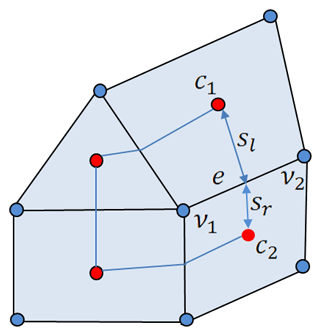Figure 1Schematic of mesh structure. Velocities are located at centroids (red circles) and elevation at vertices (blue circles). A scalar control volume associated with vertex v1 is formed by connecting neighboring centroids to edge centers. The control volumes for velocity are the triangles and quads themselves. The lines passing through two neighboring centroids (e.g., c1 and c2) are broken in a general case at edge centers. Their fragments are described by the left and right vectors directed to centroids (sl and sr for edge e). Edge e is defined by its two vertices v1 and v2 and is considered to be directed to the second vertex. It is also characterized by two elements c1 and c2 to the left and to the right, respectively.

The basic structure to describe the mesh is the array of edges given by their vertices v1 and v2, and the array of two pointers c1 and c2 to the cells on the left and on the right of the edge. There is no difference between triangles, quads, or hybrid meshes in the cycles that assemble fluxes. Quads and triangles are described through four indices to the vertices forming them; in the case of triangles the fourth index equals the first one. The treatment of triangles and quads differs slightly in computations of gradients as detailed below. We will use symbolic notation e(c) for the list of edges forming cell c, e(v) for the list of edges connected to vertex v, and v(c) for the list of vertices defining cell of element c.

In the vertical direction we introduce a σ coordinate (Phillips1957):

$\mathit{\sigma }=\frac{z+h}{h+\mathit{\zeta }},\phantom{\rule{0.25em}{0ex}}\phantom{\rule{0.25em}{0ex}}\phantom{\rule{0.25em}{0ex}}\mathrm{1}\le \mathit{\sigma }\le \mathrm{0}.$

The lower and upper horizontal faces correspond to the planes σ=0 and σ=1, respectively. The vertical grid spacing is defined by the selected set of σi. The spacing of σi is horizontally uniform in the present implementation (but it can be varying) and can be selected as equidistant or based on a parabolic function with high vertical resolution near the surface and bottom in the vertical,

${\mathit{\sigma }}_{i}=-{\left(\frac{i-\mathrm{1}}{N-\mathrm{1}}\right)}^{\mathit{\varrho }}+\mathrm{1},$

where N is the number of vertical layers. Here ϱ=1(2) gives the uniform (parabolic) distribution of vertical layers. One more possibility to use refined resolution near the bottom or surface is implemented through the formula by :

${\mathit{\sigma }}_{i}=\frac{\mathrm{tanh}\left[\left({L}_{h}+{L}_{\mathit{\zeta }}\right)\frac{\left(N-i\right)}{\left(N-\mathrm{1}\right)}-{L}_{h}\right]+\mathrm{tanh}{L}_{h}}{\mathrm{tanh}{L}_{h}+\mathrm{tanh}{L}_{\mathit{\zeta }}}-\mathrm{1},$

where Lh and Lζ are the number of layers near the bottom and surface, respectively.

The vertical grid spacing is recalculated on each baroclinic time step for the vertices where ζ is defined. It is interpolated from vertices to cells and to edges. The vectors of horizontal velocity and tracers are located in the middle of vertical layers (index $i+\mathrm{1}/\mathrm{2}$), but the vertical velocity is at full layers.

The divergence operator on scalar control volumes is computed as

$\underset{v}{\int }\mathrm{\nabla }\cdot \left(\mathrm{△}\mathbit{u}\right)\mathrm{d}S=\sum _{e=e\left(v\right)}\left[{\left(\mathrm{△}\mathbit{u}\mathbit{n}\mathrm{\ell }\right)}_{\mathrm{l}}+{\left(\mathrm{△}\mathbit{u}\mathbit{n}\mathrm{\ell }\right)}_{\mathrm{r}}\right],$

where the cycle is over edges containing vertex v, the indices l and r imply that the estimates are made on the left and right segments of the control volume boundary attached to the center of edge e, n is the outer normal, and the length of the segment (see Fig. 1). With vectors sl and sr connecting the midpoint of edge e with the cell centers on the left and on the right, we get $\left(\mathbit{n}\mathrm{\ell }{\right)}_{\mathrm{l}}=\mathbit{k}×{\mathbit{s}}_{\mathrm{l}}$ and similar, but with the minus sign for the right element (k is a unit vertical vector). The mean cell values, for example layer thickness on the cell, can be defined as ${\mathrm{△}}_{\mathrm{c}}={\sum }_{v=v\left(c\right)}{\mathrm{△}}_{v}{w}_{\mathrm{cv}}$, where ${w}_{\mathrm{cv}}=\mathrm{1}/\mathrm{3}$ on triangles and ${w}_{\mathrm{cv}}={S}_{\mathrm{cv}}/{S}_{\mathrm{c}}$ for quads (Sc cell area and Scv, the part of it in the scalar control volume around the vertex).

Gradients of scalar quantities are needed on cells and are computed as

$\underset{c}{\int }\mathrm{\nabla }\mathit{\zeta }\mathrm{d}S=\sum _{e=e\left(c\right)}{\left(\mathbit{n}\mathrm{\ell }\mathit{\zeta }\right)}_{e},$

where summation is over the edges of cell c, the normal and length are related to the edges, and ζ is estimated as the mean over edge vertices.

The gradients of velocities on cells can be needed for the computation of viscosity and the momentum advection term. They are computed through the least squares fit based on the velocities on neighboring cells:

$\mathfrak{L}=\sum _{n=n\left(c\right)}{\left({\mathbit{u}}_{c}-{\mathbit{u}}_{n}-\left({\mathit{\alpha }}_{x},{\mathit{\alpha }}_{y}\right){\mathbit{r}}_{\mathrm{cn}}\right)}^{\mathrm{2}}=min.$

Here ${\mathbit{r}}_{\mathrm{cn}}=\left({x}_{\mathrm{cn}},{y}_{\mathrm{cn}}\right)$ is the vector connecting the center of c to that of its neighbor n. Their solution can be reformulated in terms of two matrices (computed once and stored) with coefficients ${a}_{\mathrm{cn}}^{x}=\left({x}_{\mathrm{cn}}{Y}^{\mathrm{2}}-{y}_{\mathrm{cn}}XY\right)/d$ and ${a}_{\mathrm{cn}}^{y}=\left({y}_{\mathrm{cn}}{X}^{\mathrm{2}}-{x}_{\mathrm{cn}}XY\right)/d$, acting on velocity differences and returning the derivatives. Here $d={X}^{\mathrm{2}}{Y}^{\mathrm{2}}-\left(XY{\right)}^{\mathrm{2}}$, ${X}^{\mathrm{2}}={\sum }_{n=n\left(c\right)}{x}_{\mathrm{cn}}^{\mathrm{2}}$, ${Y}^{\mathrm{2}}={\sum }_{n=n\left(c\right)}{y}_{\mathrm{cn}}^{\mathrm{2}}$, and $XY={\sum }_{n=n\left(c\right)}{x}_{\mathrm{cn}}{y}_{\mathrm{cn}}$.

We implemented two options for horizontal momentum advection in the flux form. The first one is linear reconstruction upwind based on cell control volumes (see Fig. 1). The second one is central and is based on scalar control volumes, with subsequent averaging to cells. In the upwind implementation we write

$\underset{c}{\int }\mathrm{\nabla }\cdot \left(\mathbit{u}\mathrm{△}\mathbit{u}\right)\mathrm{d}S=\sum _{e=e\left(c\right)}{\left(\mathbit{u}\mathbit{n}\mathrm{\ell }\mathrm{△}\mathbit{u}\right)}_{e}.$

For edge e, linear velocity reconstructions on the elements on its two sides are estimated at the edge center. One of the cells is c, and let n be its neighbor across e. The respective velocity estimates will be denoted as uce and une, and upwind will be written in the form $\mathrm{2}\mathbit{u}={\mathbit{u}}_{\mathrm{ce}}\left(\mathrm{1}+\text{sgn}\left(\mathbit{u}\mathbit{n}\right)\right)+{\mathbit{u}}_{\mathrm{ne}}\left(\mathrm{1}-\text{sgn}\left(\mathbit{u}\mathbit{n}\right)\right)$, where $\mathbit{u}\mathbit{n}=\mathbit{n}\left(\left({\mathbit{u}}_{\mathrm{ce}}+{\mathbit{u}}_{\mathrm{ne}}\right)\right)/\mathrm{2}$.

The other form is adapted from . It provides additional smoothing for momentum advection by computing flux divergence for larger control volumes. In this case we first estimate the momentum flux term on scalar control volumes:

$\underset{v}{\int }\mathrm{\nabla }\cdot \left(\mathbit{u}\mathrm{△}\mathbit{u}\right)\mathrm{d}S=\sum _{e=e\left(v\right)}\left[{\left(\mathbit{u}\mathbit{n}\mathrm{\ell }\mathrm{△}\mathbit{u}\right)}_{\mathrm{l}}+{\left(\mathbit{u}\mathbit{n}\mathrm{\ell }\mathrm{△}\mathbit{u}\right)}_{\mathrm{r}}\right].$

The notation here follows that for the divergence. No velocity reconstruction is involved. These estimates are then averaged to the centers of cells. In both variants of advection form the fluid thickness is estimated at cell centers.

Horizontal advection and diffusion terms are discretized explicitly in time. Three advection schemes have been implemented. The first two are based on linear reconstruction for control volume and are therefore second order. The linear reconstruction upwind scheme and the Miura scheme (Miura2007) differ in the implementation of time stepping. The first needs the Adams–Bashforth method to be second order with respect to time. The scheme by Miura reaches this by estimating the tracer at a point displaced by uτ3-D∕2. In both cases a linear reconstruction of the tracer field for each scalar control volume is performed:

${\mathrm{\Theta }}_{R}\left(x,y\right)={\mathrm{\Theta }}_{\mathrm{0}}\left({x}_{v},{y}_{v}\right)+{\mathrm{\Theta }}_{x}\left(x-{x}_{v}\right)+{\mathrm{\Theta }}_{y}\left(y-{y}_{v}\right),$

where Θ0 is the tracer value at vertex, Θx and Θy are the gradients averaged to vertex locations, and xv and yv the coordinates of vertex v. The fluxes for scalar control volume faces associated with edge e are computed as

$\sum _{e=e\left(v\right)}\left(\left[{\left(\mathbit{u}\mathbit{n}\mathrm{\ell }\mathrm{△}{\mathrm{\Theta }}_{R}\right)}_{\mathrm{l}}+{\left(\mathbit{u}\mathbit{n}\mathrm{\ell }\mathrm{△}{\mathrm{\Theta }}_{R}\right)}_{\mathrm{r}}\right]\right).$

The estimate of the tracer is made at the midpoints of the left and right segments and at points displaced by uτ3-D∕2 from them, respectively.

The third approach used in the model is based on the gradient reconstruction. The idea of this approach is to estimate the tracer at mid-edge locations with a linear reconstruction using the combination of centered and upwind gradients: ${\mathrm{\Theta }}_{e}^{±}={\mathrm{\Theta }}_{{v}_{i}}±{\mathrm{\ell }}_{e}\left(\mathrm{\nabla }\mathrm{\Theta }{\right)}_{e}^{±}/\mathrm{2}$, where $i=\mathrm{1},\mathrm{2}$ are the indices of edge vertices, and gradients are computed as

$\begin{array}{ll}& \left(\mathrm{\nabla }\mathrm{\Theta }{\right)}_{e}^{+}=\frac{\mathrm{2}}{\mathrm{3}}\left(\mathrm{\nabla }\mathrm{\Theta }{\right)}^{\mathrm{c}}+\frac{\mathrm{1}}{\mathrm{3}}\left(\mathrm{\nabla }\mathrm{\Theta }{\right)}^{\mathrm{u}}\phantom{\rule{0.25em}{0ex}}\phantom{\rule{0.25em}{0ex}}\phantom{\rule{0.25em}{0ex}}\text{and}\\ & \left(\mathrm{\nabla }\mathrm{\Theta }{\right)}_{e}^{-}=\frac{\mathrm{2}}{\mathrm{3}}\left(\mathrm{\nabla }\mathrm{\Theta }{\right)}^{\mathrm{c}}+\frac{\mathrm{1}}{\mathrm{3}}\left(\mathrm{\nabla }\mathrm{\Theta }{\right)}^{\mathrm{d}}.\end{array}$

Here, the upper index c means centered estimates, and u and d imply the estimates on the up- and down-edge cells.

The advective flux of scalar quantity Θ through the face of the scalar volume $\left({Q}_{e}=\left[{\left(\mathbit{u}\mathbit{n}\mathrm{\ell }\mathrm{△}\right)}_{\mathrm{l}}+{\left(\mathbit{u}\mathbit{n}\mathrm{\ell }\mathrm{△}\right)}_{\mathrm{r}}\right]\right)$ associated with edge e, which leaves the control volume ν1 (see Fig. 1), is

${Q}_{e}{\mathrm{\Theta }}_{e}=\frac{\mathrm{1}}{\mathrm{2}}{Q}_{e}\left({\mathrm{\Theta }}_{e}^{+}+{\mathrm{\Theta }}_{e}^{-}\right)+\frac{\mathrm{1}}{\mathrm{2}}\left(\mathrm{1}-\mathit{\gamma }\right)\left|{Q}_{e}\right|\left({\mathrm{\Theta }}_{e}^{+}+{\mathrm{\Theta }}_{e}^{-}\right),$

where γ is the parameter controlling the upwind dissipation. Taking γ=0 gives the third-order upwind method, whereas γ=1 gives the centered fourth-order estimate.

A quadratic upwind reconstruction is used in the vertical with the flux boundary conditions on surface (Eqs. 8 and 9) and zero flux at the bottom. Other options for horizontal and vertical advection, including limiters, will be introduced in the future.

The advection schemes are coded so that their order can be reduced toward the first-order upwind for a very thin water layer to increase stability in the presence of wetting and drying.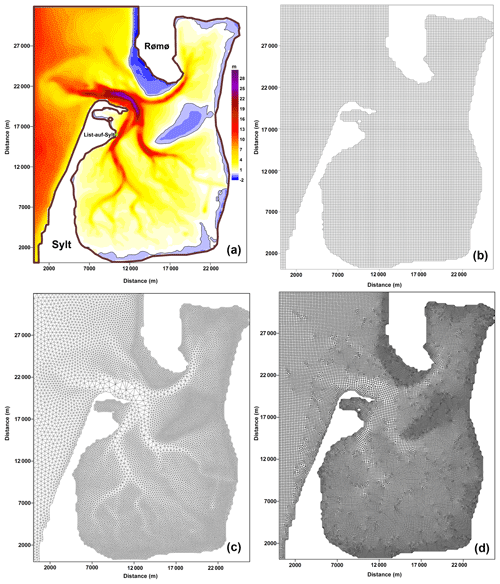Figure 2(a) The bathymetry of the Sylt–Rømø Bight (provided by Hans Burchard, personal communication, 2015) with the location of station List-auf-Sylt; (b) the regular quasi-quadrilateral MESH-1 (200 m; 16 089 vertices, 15 578 quads, and 176 triangles); (c) the triangular MESH-2 (14 193 vertices and 27 548 triangles); and (d) the irregular quadrilateral MESH-3 (35 639 vertices, 34 820 quads, and 31 triangles).Figure 3Potential and kinetic energy. Panels (a, c) are for the total area; panels (b, d) are for the area where the full depth exceeds 1 m.

## 4.4 Viscosity and filtering

Consider the operator Au. Its computation follows the rule

$\underset{c}{\int }\mathrm{\nabla }A\mathrm{\nabla }\mathbit{u}\mathrm{d}S=\sum _{e=e\left(c\right)}A\mathrm{\ell }{\left(\mathbit{n}\mathrm{\nabla }\mathbit{u}\right)}_{e}.$

The estimate of velocity gradient on edge e is symmetrized following the standard practice (Danilov2012) over the values on neighboring cells. “Symmetrized” means that the estimate on edge e is the mean of horizontal velocity gradients computed on elements c and n (notation from article) with the common edge e: $\left(\mathrm{\nabla }\mathbit{u}{\right)}_{e}=\left(\left(\mathrm{\nabla }\mathbit{u}{\right)}_{c}+\left(\mathrm{\nabla }\mathbit{u}{\right)}_{n}\right)/\mathrm{2}$. The consequence of this symmetrization is that on regular meshes (formed of equilateral triangles or rectangular quads) the information from the nearest neighbors is lost. Any irregularity in velocity on the nearest cells will not be penalized. Although unfavorable for both quads and triangles, it has far-reaching implications for the latter: it cannot efficiently remove the decoupling between the nearest velocities, which may occur for triangular cells. This fact is well known, and the modification of the scheme above that improves coupling between the nearest neighbors consists of using the identity

$\mathbit{n}={\mathbit{r}}_{\mathrm{cn}}/\left|{\mathbit{r}}_{\mathrm{cn}}\right|+\left(\mathbit{n}-{\mathbit{r}}_{\mathrm{cn}}/\left|{\mathbit{r}}_{\mathrm{cn}}\right|\right),$

where rcn is the vector connecting the centroid of cells c and n. The derivative in the direction of rcn is just the difference between the neighboring velocities divided by the distance, which is explicitly used to correct nu. It is easy to show that on rectangular quads or equilateral triangles (n and rcn are collinear) the second term of the expression above will disappear. This is the harmonic discretization and a biharmonic version is obtained by applying the procedure twice.

A simpler algorithm is implemented to control grid-scale noise in the horizontal velocity. It consists of adding to the right hand for the momentum equation (2-D and 3-D flow) a term coupling the nearest velocities,

${F}_{c}=-\left(\frac{\mathrm{1}}{{\mathit{\tau }}_{\mathrm{f}}}\right)\sum _{n\left(c\right)}\left({\mathbit{u}}_{n}-{\mathbit{u}}_{c}\right),$

where τf is a timescale selected experimentally. On regular meshes this term is equivalent to the Laplacian operator. On general meshes it deviates from the Laplacian, yet after some trivial adjustments it warrants momentum conservation and energy dissipation .

## 4.5 Wetting and drying algorithm

For modeling wetting and drying we use the method proposed by . The idea of this method is to accurately track the moving shoreline by employing the upwind water depth in the flux computations. The criterion for a vertex to be wet or dry is taken as

where Dmin is the critical depth and hl is the topography. Each cell is treated as

where hv and ζv are the depth and sea surface height at the vertices v(c) of the cell c. When a cell is treated as dry, the velocity at its center is set to zero and no volume flux passes through the boundaries of scalar control volumes inside this cell.

5 Numerical simulations

In this section we present the results of two model experiments. The first considers tidal circulation in the Sylt–Rømø Bight. This area has a complex morphometry with big zones of wetting–drying and large incoming tidal waves. In this case our intention is to test the functioning of meshes of various kinds. The second experiment simulates the southeast part of the North Sea. For this area, an annual simulation of barotropic–baroclinic dynamics with realistic boundary conditions on open and surface boundaries is carried out and compared to observations. We note that a large number of simpler experiments, including those in which analytical solutions are known, were carried out in the course of model development to test and tune the model accuracy and stability. Lessons learned from these were taken into account. We omit their discussion in favor of realistic simulations.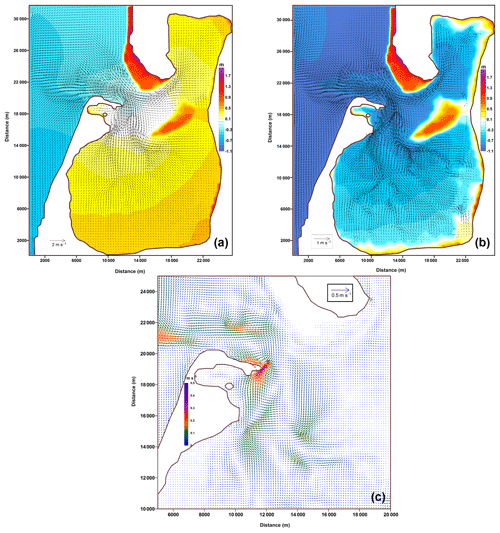Figure 4(a) Full ebb; (b) low water; (c) the residual circulation. Simulation was performed on MESH-1.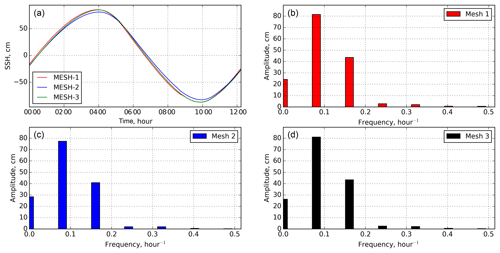Figure 5(a) Sea surface height (SSH) for one tidal period in the station List-auf-Sylt (see Fig. 2); (b) spectrum of the computed M2 tidal sea level at station List-auf-Sylt on MESH-1; (c) spectrum on MESH-2; and (d) spectrum on MESH-3.

## 5.1 Sylt–Rømø experiment

To test the code sensitivity to the type of grid and grid quality, we computed barotropic tidally driven circulation in the Sylt–Rømø Bight in the Wadden Sea.

It is a popular area for experiments and test cases . The Sylt and Rømø islands are connected to the mainland by artificial dams, creating a relatively small semi-enclosed bight with a circulation pattern well known from observations and modeling (e.g., Becherer et al.2011; Purkiani et al.2014). It is a tidally energetic region with a water depth down to 30 m, characterized by wide intertidal flats and a rugged coastline. Water is exchanged with the open sea through a relatively narrow (up to 1.5 km wide) and deep (up to 30 m) tidal inlet, Lister Dyb. The bathymetry data for the area were provided by Hans Burchard (personal communication, 2015) and are presented in Fig. 2.

We constructed three different meshes (Fig. 2) for our experiments. The first one is a nearly regular quadrilateral mesh, complemented by triangles that straighten the coastline (MESH-1). Its spatial resolution is 200 m. The second mesh is purely triangular (MESH-2) with resolution varying from ∼820 to ∼90 m. The third mesh was generated by the Gmsh mesh generator and includes 34 820 quads and 31 triangles with a minimum cell size of 30 m and maximum size of ∼260 m (MESH-3). All meshes have 21 nonuniform sigma layers in the vertical direction (refined near the surface and bottom). The wetting–drying option is turned on. We apply the kϵ turbulence closure model with transport equations for the turbulent kinetic energy and the turbulence dissipation rate using the GOTM library. The second-moment closure is represented by algebraic relations suggested by . The experiment is forced by prescribing elevation due to an M2 tidal wave at the open boundary (western and northern boundaries of the domain) provided by Hans Burchard (personal communication, 2015).

Simulations on each mesh were continued until reaching the steady state in the tidal cycle of the M2 wave. The last tidal period was analyzed. Quasi-stationary behavior is already established in the second tidal period. The simulated M2 wave is essentially nonlinear during the tidal cycle judged by the difference in amplitude of two tidal half-cycles.

Figure 3 shows the behavior of potential and kinetic energies in the entire domain, and the right panels show the energies computed over the areas deeper than 1 m. The results are sensitive to the meshes, which is explained further. The smallest tidal energy is simulated on the triangular mesh (MESH-2). The reason is that with the same value of the timescale τf in the filter used by us in these simulations, the effective viscous dissipation is much higher on a triangular mesh than on quadrilateral meshes of similar resolution. However, the solutions on quadrilateral meshes are also different, and this time the reason is the difference in the details of representing very shallow areas on meshes of various resolution (MESH-3 is finer than MESH-1). The difference between the simulations on two quadrilateral meshes is related to the potential energy and comes from the difference in the elevation simulated in the areas subject to wetting and drying (see Fig. 8). Note that the velocities and layer thickness are small in these areas, so the difference between kinetic energies in Fig. 3c and d is small.

The average currents, sea level, and residual circulation simulated on MESH-1 are presented in Fig. 4. The results of this experiment show good agreement with the previously published results of .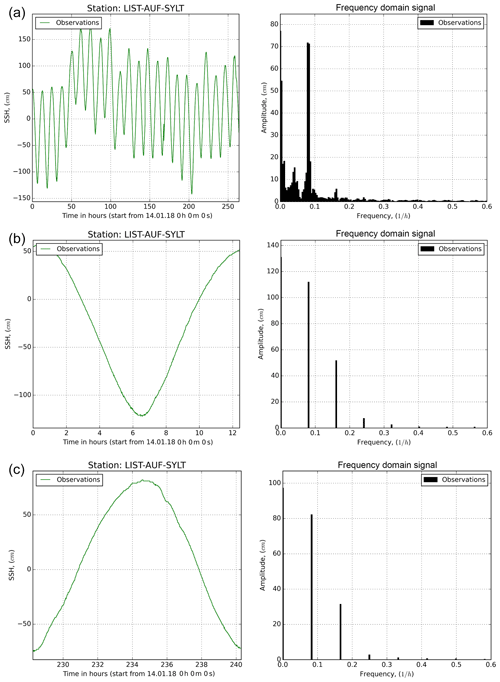Figure 6(a) Spectrum of the observation tidal sea level at station List-auf-Sylt (see Fig. 2) from 1 to 15 January 2018; (b) spectrum of the observation SSH for one tidal period with strong wind (1 January 2018); and (c) spectrum of the observation SSH for one tidal period with no wind (14 January 2018).

An example spectrum of level oscillations on station List-auf-Sylt from model results is presented in Fig. 5. The amplitude of the M2 wave on quad meshes (MESH-1 and MESH-3) slightly exceeds 80 cm and is a bit smaller on MESH-2. Similar behavior is seen for the second harmonics (M4) expressing nonlinear effects in this region. We tried to compare model simulation with the observations (https://www.pegelonline.wsv.de/gast/start, last access: 28 February 2019). For comparison, the observations were taken for the first half of January 2018. Figure 6 presents the range of fluctuations for the whole period. As is seen, the main tidal wave M2 has a smaller amplitude (about 70 cm) than in simulations. However, the high-frequency part of the spectrum is very noisy because of atmospheric loading and winds. If the analysis is performed for separate tidal cycles in cases of strong wind and no wind, the correspondence with observations is recovered in the second case.

Of particular interest is the convergence of the solution on different meshes. For comparison the solutions simulated on MESH-2 and MESH-3 were interpolated to MESH-1. The comparison was performed for the full tidal cycle and is shown in Fig. 7, which presents histograms of the differences.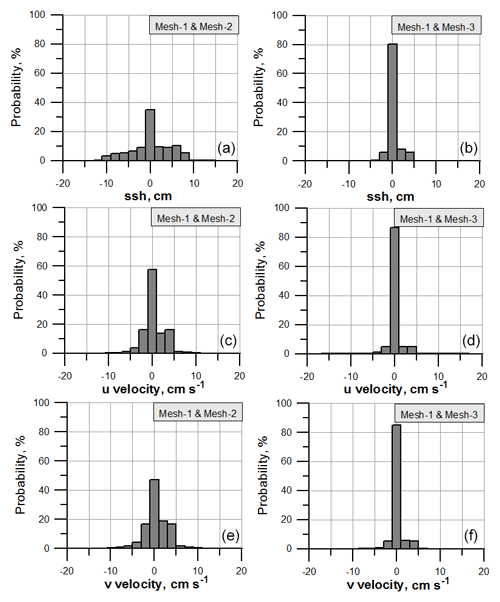Figure 7Histograms of the difference between solutions for the tidal cycle of the M2 wave on MESH-1 and MESH-2 (a, c, e) and on MESH-1 and MESH-3 (b, d, f). Top, middle, and bottom rows correspond to the difference in elevation and the u and v components of velocity, respectively.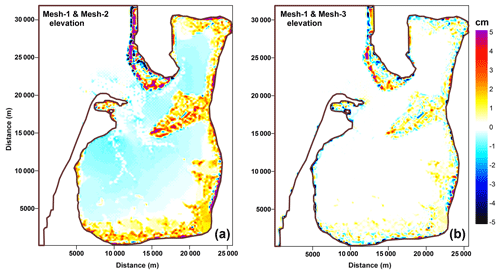Figure 8Spatial distribution of the elevation differences for a full tidal period for MESH-1 and MESH-2 (a) and for MESH-1 and MESH-3 (b).

For the solutions on MESH-1 and MESH-3 values at more than 80 % of points agree within the range of ±1 cm for the elevation (the maximum tidal wave exceeds 1 m) and within the range of ±1 cm s−1 for the velocity (the maximum horizontal velocity is about 120 cm s−1). Thus, the agreement between simulations on quadrilateral MESH-1 and MESH-3 is also maintained on a local level. The agreement becomes worse when comparing solutions on triangular MESH-2 and quadrilateral MESH-1. Here the share of points with larger deviations is noticeably larger.

Spatial patterns of the differences for elevations and velocities simulated on different meshes are presented in Figs. 8 and 9, respectively. Substantial differences for the elevation are located in wetting and drying zones. This is related to the sensitivity of the wetting and drying algorithm to the cell geometry. For the horizontal velocity the difference between the solutions is defined by the resolution of bottom topography in the most energetically active zone on the quadrilateral meshes (see residual circulation in Fig. 4). The difference between the triangular grid and quadrilateral grid has a noisy character and is seen in the regions of strongest depth gradients.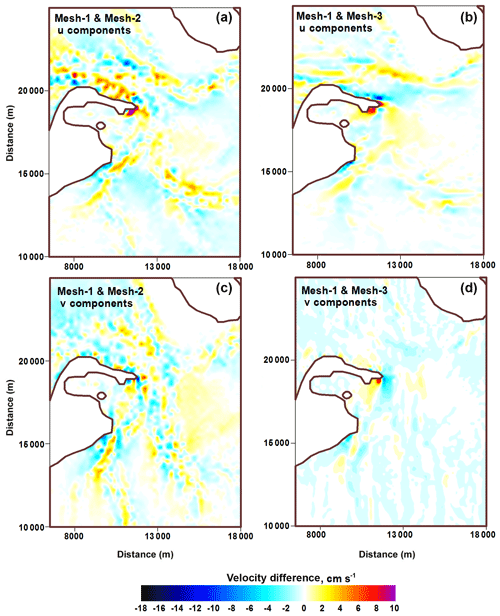Figure 9Spatial distribution of the difference between the horizontal velocities for the full tidal period of an M2 wave: u component (a, b); v component (c, d). The differences are between MESH-1 and MESH-2 (a, c) and MESH-1 and MESH-3 (b, d).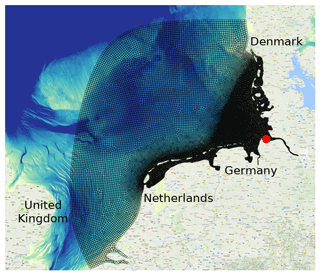Figure 10The area of the southeast North Sea experiment with mesh (black lines). The red dot indicates the position of the Cuxhaven station. This mesh includes 31 406 quads and 32 triangles.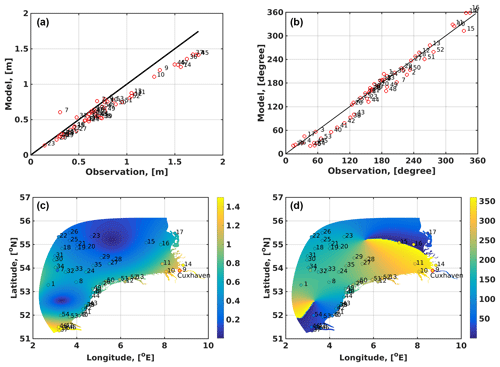Figure 11The simulated M2 tidal map in the south North Sea experiment compared to observations. The amplitude is in meters (a, c) and phase (b, d) in degrees. (a, b) Model-to-observation graphs; the numbers correspond to station numbers shown in (c, d). The color shows the amplitude in (a) and phase in (b), and the filled circles show the observational data. The red circle indicates the position of Cuxhaven station. The total vector error is 0.24 m for 53 stations.Figure 12Modeled (blue line) and observed (gray dots and dashed black lines) sea surface salinity (SSS) at the Cuxhaven station. The station is positioned at the mouth of the Elbe River between stations 9 and 13 in Fig. 11. Panel (a) shows 9 months of simulations, and panel (b) shows results from two selected days in May. The blue (modeled with the Miura advection scheme) and thick dashed black (observation) lines in (a) show running-mean SSS with a time window of 10 periods for the M2 tidal wave. Thin dashed black lines are 1 standard deviation bounds of the running-mean observed SSS in (a).

## 5.2 Southeast North Sea circulation

Here we present the results of realistic simulations of circulation in the southeastern part of the North Sea. The area of simulations is limited by the Dogger Bank and Horns Rev (Denmark) on the north and the border between Belgium and the Netherlands on the west. It is characterized by complex bathymetry with strong tidal dynamics . The related estuarine circulation , strong lateral salinity, and nutrient gradients and river plumes are important aspects of this area. In our simulations, the mesh consists of mainly quadrilateral cells. The mesh is constructed with Gmsh using the Blossom-Quad method . It includes 31 406 quads and only 32 triangles. The mesh resolution (defined as the distance between vertices) varies between 0.5 and 1 km in the area close to the coast and Elbe estuary, coarsening to and 4–5 km at the open boundary (Fig. 10). The mesh contains five sigma layers in the vertical.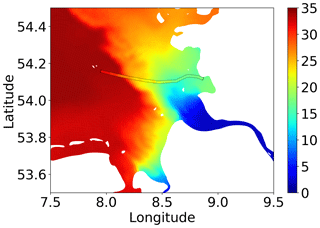Figure 13Sea surface salinity on 26 June 2013. Filled contours are model results, and colored lines are observational data from FerryBox (FunnyGirl) (Petersen2014).

Bathymetry from the EMODnet Bathymetry Consortium (2016) has been used. Model runs were forced by 6-hourly atmospheric data from NCEP/NCAR Reanalysis and daily resolved observed river runoff . Salinity and temperature data on the open boundary were extracted from hindcast simulations based on TRIM-NP . The sea surface elevation at the open boundary was prescribed in terms of amplitudes and phase for M2 and M4 tidal waves derived from the previous simulations of the North Sea . Data for temperature and salinity from the TRIM-NP model were used to initialize model runs for 1 year. The results of these runs were used as initial conditions for a 10-month final simulation.

The validation of simulated amplitudes and phases of the M2 tidal wave is presented in Fig. 11. This wave is the main tidal constituent in this region. It enters the domain at the western boundary and propagates along the coast as a Kelvin wave. The phase field is characterized by two amphidromic points. We used the observed values from Ole Baltazar Andersen (personal communication, 2008). for the comparison. The simulated amplitudes are generally slightly smaller than the observed ones (Fig. 11). The deviations in amplitudes can be explained by uncertainty in model bathymetry and the use of a constant bottom friction coefficient. The phases of the M2 wave are well reproduced by the model. We characterize its accuracy by the total vector error:

$\mathit{\mu }=\frac{\mathrm{1}}{N}\sum _{n=\mathrm{1}}^{N}{\left({\left({A}_{*}\mathrm{cos}{\mathit{\phi }}_{*}-A\mathrm{cos}\mathit{\phi }\right)}^{\mathrm{2}}+{\left({A}_{*}\mathrm{sin}{\mathit{\phi }}_{*}-A\mathrm{sin}\mathit{\phi }\right)}^{\mathrm{2}}\right)}_{n}^{\mathrm{1}/\mathrm{2}},$

where A*, φ* and A, φ are the observed and computed amplitudes and phases, respectively, at N stations. The total vector error is 0.24 m for 53 stations in the entire simulated domain, which presents a reasonably good result for this region given the domain size. From the results of comparison it is seen that observations at some stations, such as station 7 in the open sea, differ considerably from the amplitude and phase at the close stations. The comparison will improve if such outlier stations are excluded.

To validate the simulated temperature and salinity we used data from the COSYNA database and ICES database (http://www.ices.dk, last access: 28 February 2019). Comparisons of modeled surface temperature and salinity show good Pearson correlation coefficients of 0.98 and 0.9 with RMSD values of 1.24 and 0.98, respectively. The model can represent both seasonal changes in sea surface temperature (SST) and salinity (SSS), as well as lateral gradients (not shown) reasonably well. The modeled and observed SSS for Cuxhaven station is presented in Fig. 12 for simulations with the Miura advection scheme.

The observations are from the station located in the mouth of the Elbe River near the coast. They are characterized by a tidal amplitude in excess of 1.5 m, a horizontal salinity gradient of 0.35 PSU km−1 (during spring tide up to 0.45 PSU km−1) (https://www.portal-tideelbe.de, last access: 28 February 2019, and ), and an extended wetting and drying area around this station. The simulation is in good agreement with tidal filtered mean SSS (Fig. 12). The model represents the summer flood event during June–July well.

Figure 13 shows the calculated surface salinity field in part of the simulated domain on 26 June 2013 in comparison with the observational data from FerryBox (FunnyGirl) (Petersen2014). As can be seen from the plot, there is a high consistency of the simulated results with observational data.

6 Discussion

## 6.1 Triangles vs. quads: numerical performance

We examine the computational efficiency by comparing the CPU time needed to simulate five tidal periods of an M2 wave on MESH-1 and MESH-2 in the Sylt–Rømø experiment, as presented in Fig. 14. The number of vertices of the quadrilateral MESH-1 is approximately ∼1.13 that of triangular MESH-2, but the numbers of elements relate as ∼0.57. We have found that the total CPU times are in an approximate ratio of 1.62 (triangles  quads). The simulations were performed with the same time steps.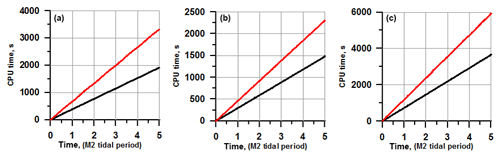Figure 14CPU time on two meshes, MESH-1 (black line) and MESH-2 (red line), for the Sylt–Rømø experiment. The CPU time for 3-D velocity (a), external mode (b), and the total CPU time (c).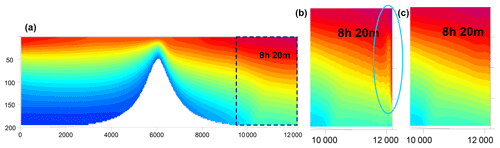Figure 15Temperature section along the channel as simulated on the quadrilateral mesh (a). The two inserts show the area adjacent to the open boundary on the purely triangular mesh (a) and the mesh for which the vicinity of the open boundary is rendered with quads (c). The dashed rectangle shows the area of the inserts. Numerical instability evolves on a purely triangular mesh (blue ellipse).

The 3-D velocity part takes approximately the same CPU time as the computation of vertically averaged velocity and elevation (external mode). Operations on elements, which include the Coriolis and bottom friction terms as well as computations of the gradients of velocity and scalars, are approximately twice as cheap on quadrilateral meshes as expected. Computations of viscosity and momentum transport are carried out in a cycle over edges, which is 1.5 times shorter for meshes made of quadrilateral elements and warrants a similar gain of ∼1.5 in performance on quadrilateral meshes. In our simulation, the net gain was ∼1.62 times on MESH-1 compared to MESH-2, despite the fact that the number of vertices is 13 % larger than on MESH-2. The model is stable on the quadrilateral meshes with smaller horizontal viscosity, which is also an advantage.

## 6.2 Triangles vs. quads: open boundaries

The presence of open boundaries is a distinctive feature of regional models. The implementation of robust algorithms for the open boundary is more complicated on unstructured triangular meshes than on structured quadrilateral meshes. For example, it is more difficult to cleanly assess the propagation of perturbations toward the boundary in this case. In addition, spurious inertial modes can be excited on triangular meshes in the case of the cell-vertex discretization used by us, which in practice leads to additional instabilities in the vicinity of the open boundary. The ability to use hybrid meshes is very helpful in this case. Indeed, even if the mesh is predominantly triangular, the vicinity of the open boundary can be constructed of quadrilateral elements.

We illustrate improvements of the dynamics in the vicinity of the open boundary by simulating baroclinic tidal dynamics in an idealized channel with an underwater sill. The channel is 12 km in length and 3 km in width, with a maximum depth of 200 m near the open boundary. The sill, with a height of 150 m, is located in the central part of the channel. The flow is forced at the open boundaries by a tide with the period of an M2 wave and amplitude of 1 cm, applied in antiphase. The left part of the channel contains denser waters than the right one.

Three meshes were used for these simulations. The first one is a quadrilateral mesh with a horizontal resolution of 200 m refined to 20 m in the vicinity of the underwater sill. The second one is a purely triangular mesh obtained from the quadrilateral mesh by splitting quads into triangles. The third mesh is predominantly triangular, but for the zones close to the open boundary it is also quadrilateral.

Figure 15 illustrates that at times close to the maximum inflow (8 h 20 m), a strong computational instability due to the interaction between baroclinic and barotropic flow components evolves on the right open boundary on the triangular mesh, eventually leading to the blowup of the solution (see the left insert). However, by replacing triangles in a small domain adjacent to the open boundary with quadrilateral cells we stabilize the numerical solution (see the right insert), which allows us to cleanly handle the directions normal and tangent to the boundary.

7 Conclusions

We described the numerical implementation of the three-dimensional unstructured-mesh model FESOM-C, relying on FESOM2 and intended for coastal simulations. The model is based on a finite-volume cell-vertex discretization and works on hybrid unstructured meshes composed of triangles and quads.

We illustrated the model performance with two test simulations.

Sylt–Rømø Bight is a closed Wadden Sea basin characterized by a complex morphometry and high tidal activity. A sensitivity study was carried out to elucidate the dependence of simulated surface elevation and horizontal velocity on mesh type and quality. The elevation simulated in zones of wetting and drying may depend on the mesh structure, which may lead to distinctions in the simulated energy on different meshes. The total energy comparison shows that on the triangular MESH-2, having approximately the same number of vertices as MESH-1, the solution is more dissipative; higher dissipation is generally needed to stabilize it against spurious inertial modes.

The second experiment deals with the southeastern part of the North Sea. Computation relied on the boundary information from hindcast simulations by the TRIM-NP and realistic atmospheric forcing from NCEP/NCAR. Modeling results agree both qualitatively and quantitatively with observations for the full period of simulation.

Future development of the FESOM-C will include coupling with the global FESOM2 , the addition of monotonic high-order schemes and sea ice from FESOM2, and various modules that would increase the functionality of FESOM-C.

Code and data availability

The version of FESOM-C v.2 used to carry out simulations reported here can be accessed from https://doi.org/10.5281/zenodo.2085177. The General Ocean Turbulence Model (GOTM) implemented into the FESOM-C code is published under the GNU Public License and can be freely used. The meshes are constructed with the Gmsh software . Bathymetry used in the model simulation (Southeast North Sea experiment) is received from the EMODnet Bathymetry Consortium (2016) database (https://doi.org/10.12770/c7b53704-999d-4721-b1a3-04ec60c87238) and is freely available online. The TRIM-NP model is used to initialize runs for 1 year . NCEP/NCAR reanalysis atmospheric forcing data used in the model are freely available online.

Author contributions

AA is the developer of the FESOM-C model with support from VF, IK, and SD. VF, IK, and AA carried out the experiment. AA wrote the paper with support from SD, VF, and IK. SH and NR carried out the code optimization and parallelization. KHW and HB contributed with discussions of many preliminary results. KHW helped supervise the project. All authors discussed the results and commented on the paper at all stages.

Competing interests

The authors declare that they have no conflict of interest.

Acknowledgements

We are grateful to Jens Schröter, Wolfgang Hiller, Peter Lemke, and Thomas Jung for supporting our work.

The article processing charges for this open-access
publication were covered by a Research
Centre of the Helmholtz Association.

Edited by: Robert Marsh
Reviewed by: two anonymous referee

References

Androsov, A. A., Klevanny, K. A., Salusti, E. S., and Voltzinger, N. E.: Open boundary conditions for horizontal 2-D curvilinear-grid long-wave dynamics of a strait, Adv. Water Resour., 18, 267–276, https://doi.org/10.1016/0309-1708(95)00017-D, 1995. a

Barnier, B., Siefridt, L., and Marchesiello, P.: Thermal forcing for a global ocean circulation model using a three-year climatology of ECMWF analyses, J. Marine Syst., 6, 363–380, https://doi.org/10.1016/0924-7963(94)00034-9, 1995. a

Baschek, B., Schroeder, F., Brix, H., Riethmüller, R., Badewien, T. H., Breitbach, G., Brügge, B., Colijn, F., Doerffer, R., Eschenbach, C., Friedrich, J., Fischer, P., Garthe, S., Horstmann, J., Krasemann, H., Metfies, K., Merckelbach, L., Ohle, N., Petersen, W., Pröfrock, D., Röttgers, R., Schlüter, M., Schulz, J., Schulz-Stellenfleth, J., Stanev, E., Staneva, J., Winter, C., Wirtz, K., Wollschläger, J., Zielinski, O., and Ziemer, F.: The Coastal Observing System for Northern and Arctic Seas (COSYNA), Ocean Sci., 13, 379–410, https://doi.org/10.5194/os-13-379-2017, 2017. a

Becherer, J., Burchard, H., Floeser, G., Mohrholz, V., and Umlauf, L.: Evidence of tidal straining in well-mixed channel flow from micro-structure observations, Geophys. Res. Lett., 38, L17611, https://doi.org/10.1029/2011GL049005, 2011. a

Benjamin, T. B.: Gravity currents and related phenomena, J. Fluid Mech., 31, 209–248, 1968.

Blumberg, A. F. and Mellor, G. L.: A Description of a three-dimensional coastal ocean circulation model, in: Three-dimensional Coastal Ocean Models, edited by: Mooers, C. N. K., Coast. Est. S., 4, 1–16, 1987. a, b, c

Burchard, H., Bolding, K., and Ruiz-Villarreal, M.,: GOTM, a general ocean turbulence model. Theory, implementation and test cases, European Commission, Tech. Rep. EUR 18745 EN, 1999. a, b

Burchard, H. and Bolding, K.: GETM, a general estuarine transport model. Scientific documentation, European Commission, Tech. Rep. EUR 20253 EN, 2002. a

Burchard, H., Flöser, G., Staneva, J. V., Badewien, T. H., and Riethmüller, R.: Impact of Density Gradients on Net Sediment Transport into the Wadden Sea, J. Phys. Oceanogr., 38, 566–587, https://doi.org/10.1175/2007JPO3796.1, 2008. a, b

Casulli, V. and Walters, R. A.: An unstructured grid, three-dimensional model based on the shallow water equations, Int. J. Numer. Meth. Fl., 32, 331–348, 2000. a

Cebeci, T. and Smith, A. M. O.: Analysis of Turbulent Boundary Layers, Academic Press, New York, 1974. a

Chen, C., Liu, H., and Beardsley, R. C.: An unstructured, finite volume, three-dimensional, primitive equation ocean model: application to coastal ocean and estuaries, J. Atmos. Ocean. Tech., 20, 159–186, 2003. a, b

Cheng, Y., Canuto, V. M., and Howard, A. M.: An improved model for the turbulent PBL, J. Atmos. Sci., 59, 1550–1565, 2002. a

Danilov, S.: Two finite-volume unstructured mesh models for large-scale ocean modeling, Ocean Model., 47, 14–25, https://doi.org/10.1016/j.ocemod.2012.01.004, 2012. a, b

Danilov, S. and Androsov, A.: Cell-vertex discretization of shallow water equations on mixed unstructured meshes, Ocean Dynam., 65, 33–47, https://doi.org/10.1007/s10236-014-0790-x, 2015. a, b, c

Danilov, S., Sidorenko, D., Wang, Q., and Jung, T.: The Finite-volumE Sea ice–Ocean Model (FESOM2), Geosci. Model Dev., 10, 765–789, https://doi.org/10.5194/gmd-10-765-2017, 2017. a, b, c

Deleersnijder, E. and Roland, M.: Preliminary tests of a hybrid numerical-asymptotic method for solving nonlinear advection-diffusion equations in a domain limited by a selfadjusting boundary, Math. Comput. Model., 17, 35–47, 1993. a

Deleersnijder, E.: Numerical mass conservation in a free-surface sigma coordinate marine model with mode splitting, J. Marine Syst., 4, 365–370, 1993. a

Flöser, G., Burchard, H., and Riethmüller, R.: Observational evidence for estuarine circulation in the German Wadden Sea, Cont. Shelf Res., 31, 1633–1639, 2011. a

Fringer, O. B., Gerritsen, M., and Street, R. L.: An unstructured-grid, finite-volume, nonhydrostatic, parallel coastal ocean simulator, Ocean Model., 14, 139–173, 2006. a

Gadd, A. J.: A split explicit integration scheme for numerical weather prediction, Q. J. Roy. Meteor. Soc., 104, 569–582, https://doi.org/10.1002/qj.49710444103, 1978. a

Geuzaine, C. and Remacle, J.-F.: Gmsh: a three-dimensional finite element mesh generator with built-in pre- and post-processing facilities, Int. J. Numer. Meth. Eng., 79, 1309–1331, 2009. a, b, c

Higdon, R. L.: A comparison of two formulations of barotropic-baroclinic splitting for layered models of ocean circulation, Ocean Model., 24, 29–45, 2008. a

Idier, D., Paris, F., Cozannet, G. L., Boulahya, F., and Dumas, F.: Sea-level rise impacts on the tides of the European Shelf, Cont. Shelf Res., 137, 56–71, 2017. a

Kalnay, E., Kanamitsu, M., Kistler, R., Collins, W., Deaven, D., Gandin, L., Iredell, M., Saha, S., White, G., Woollen, J., Zhu, Y., Leetmaa, A., Reynolds, B., Chelliah, M., Ebisuzaki, W., Higgins, W., Janowiak, J., Mo, K. C., Ropelewski, C., Wang, J., Jenne, R., and Joseph, D.: The NCEP/NCAR 40-Year Reanalysis Project. B. Am. Meteorol. Soc., 77, 437–472, 1996. a, b

Kappenberg, J., Berendt, M., Ohle, N., Riethmuller, R., Schuster, D., and Strotmann, T.: Variation of Hydrodynamics and Water Constituents in the Mouth of the Elbe Estuary, Germany, Civil Eng. Res. J., 4, 555643, https://doi.org/10.19080/CERJ.2018.04.555643, 2018. a

Kerimoglu, O., Hofmeister, R., Maerz, J., Riethmüller, R., and Wirtz, K. W.: The acclimative biogeochemical model of the southern North Sea, Biogeosciences, 14, 4499–4531, https://doi.org/10.5194/bg-14-4499-2017, 2017. a

Lazure, P. and Dumas F.: An external–internal mode coupling for a 3D hydrodynamical model for applications at regional scale (MARS), Adv. Water Resour., 31, 233–250, 2008. a

Le Roux, D. Y.: Spurious inertial oscillations in shallow-water models, J. Comput. Phys., 231, 7959–7987, 2012. a

Lumborg, U. and Pejrup, M.: Modelling of cohesive sediment transport in a tidal lagoon an annual budget, Mar. Geol., 218, 1–16, 2005. a

Luyten, P. J., Jones, J. E., Proctor, R., Tabor, A., Tett P., and Wild-Allen, K., COHERENS – A coupled hydrodynamical-ecological model for regional and shelf seas: User Documentation. MUMM Report, Management Unit of the Mathematical Models of the north Sea, Belgium, 911 pp., 1999. a

Marchesiello, P., McWilliams J. C., and Shchepetkin, A.: Open boundary conditions for long-term integration of regional oceanic models, Ocean Model., 3, 1–20, https://doi.org/10.1016/S1463-5003(00)00013-5, 2001. a

Marshall, J., Hill, C., Perelman, L., and Adcroft, A.: Hydrostatic, quasi-hydrostatic, and nonhydrostatic ocean modeling, J. Geophys. Res., 102, 5733–5752, https://doi.org/10.1029/96JC02776, 1997. a

Maßmann, S., Androsov, A., and Danilov, S.: Intercomparison between finite element and finite volume approaches to model North Sea tides, Cont. Shelf Res., 30, 680–691, https://doi.org/10.1016/j.csr.2009.07.004, 2010. a, b

Miura, H.: An upwind-biased conservative advection scheme for spherical hexagonal-pentagonal grids, Mon. Weather Rev., 135, 4038–4044, 2007. a

Pätsch, J. and Lenhart, H. J.: Daily Loads of Nutrients, Total Alkalinity, Dissolved Inorganic Carbon and Dissolved Organic Carbon of the European Continental Rivers for the Years 1977–2002, Berichte aus dem Zentrum für Meeres- und Klimaforschung/B: Ozeanographie/Institut für Meereskunde, Inst. für Meereskunde, Universität Hamburg, Germany, 2011. a

Pätsch, J. and Lenhart, H. J.: Daily Loads of Nutrients, Total Alkalinity, Dissolved Inorganic Carbon and Dissolved Organic Carbon of the European Continental Rivers for the Years 1977–2002, Berichte aus dem Zentrum für Meeres- und Klimaforschung; Reihe B: Ozeanographie, 48, 159 pp., 2004.

Peterson, W.: FerryBox systems: State-of-the-art in Europe and future development, J. Marine Syst., 140, 4–12, 2014. a, b

Phillips, N. A.: A coordinate system having some special advantages for numerical forecasting, J. Meteorol., 14, 184–185, 1957. a

Purkiani, K., Becherer, J., Flöser, G., Gräwe, U., Mohrholz, V., Schuttelaars, H. M., and Burchard, H.: Numerical analysis of stratification and destratification processes in a tidally energetic inlet with an ebb tidal delta, J. Geophys. Res.-Oceans, 120, 225–243, https://doi.org/10.1002/2014JC010325, 2014. a, b

Radach, G. and Pätsch, J.: Variability of continental riverine freshwater and nutrient inputs into the North Sea for the years 1977–2000 and its consequences for the assessment of eutrophication, Estuar. Coast., 30, 66–81, 2007. a

Raymond, H. W. and Kuo, L. H.: A radiation boundary condition for multi-dimensional flows, Q. J. Roy. Meteor. Soc., 110, 535–551, https://doi.org/10.1002/qj.49711046414, 1984. a

Reid, R. O.: Modification of the quadratic bottom-stress law for turbulent channel flow in the presence of surface wind-stress, U.S. Army Corps of Engineers, Beach Erosion Board. Tech. Memo., 3, 33 pp., 1957. a

Remacle, J.-F., Lambrechts, J., Seny, B., Marchandise, E., Johnen A., and Geuzainet, C.: Blossom-Quad: A non-uniform quadrilateral mesh generator using a minimum-cost perfect-matching algorithm, Int. J. Numer. Meth. Eng., 89, 1102–1119, 2012. a

Ruiz-Villarreal, M., Bolding, K., Burchard, H., and Demirov, E.: Coupling of the GOTM turbulence module to some three-dimensional ocean models, Marine Turbulence: Theories, Observations, and Models Results of the CARTUM Project, 225–237, 2005. a, b

Shchepetkin, A. F. and McWilliams, J. C.: The Regional Ocean Modeling System: A split-explicit, free-surface, topography following coordinates ocean model, Ocean Model., 9, 347–404, 2005. a, b, c

Stelling, G. S. and Duinmeijer, S. P. A.: A staggered conservative scheme for every Froude number in rapidly varied shallow water flows, Int. J. Numer. Meth. Fl., 43, 1329–1354, https://doi.org/10.1002/fld.537, 2003. a

Umlauf, L. and Burchard, H.: Second-order turbulence closure models for geophysical boundary layers. A review of recent work, Cont. Shelf Res., 25, 795–827, 2005. a

Umlauf, L., Burchard, H., and Bolding, K.: General Ocean Turbulence Model. Scientific documentation, v3.2, Marine Science Reports 63, 274 pp., 2005. a

Umlauf, L., Burchard, H., and Bolding, K.: GOTM, Sourcecode and Test Case Documentation, Version 4.0, available at: https://gotm.net/manual/stable/pdf/a4.pdf (last access: 1 March 2019), 2007.  a

Voltzinger, N. E.: Long Waves on Shallow Water, Gidrometioizdat, Leningrad, 160 pp., 1985 (in Russian). a

Voltzinger, N. E., Klevanny, K. A., and Pelinovsky, E. N.: Long-Wave Dynamics of the Coastal Zone, Gidrometioizdat, Leningrad, 272 pp., 1989 (in Russian). a

Voynova, Y. G., Brix, H., Petersen, W., Weigelt-Krenz, S., and Scharfe, M.: Extreme flood impact on estuarine and coastal biogeochemistry: the 2013 Elbe flood, Biogeosciences, 14, 541–557, https://doi.org/10.5194/bg-14-541-2017, 2017. a

Weisse, R., Bisling, P., Gaslikova, L., Geyer, B., Groll, N., Hortamani, M., Matthias, V., Maneke, M., Meinke, I., Meyer, E., Schwichtenberg, F., Stempinski, F., Wiese, F., and Wöckner-Kluwe, K.: Climate services for marine applications in Europe, Earth Perspectives, 2, 3, https://doi.org/10.1186/s40322-015-0029-0, 2015. a, b

Wang, Q., Danilov, S., Sidorenko, D., Timmermann, R., Wekerle, C., Wang, X., Jung, T., and Schröter, J.: The Finite Element Sea Ice–Ocean Model (FESOM) v.1.4: formulation of an ocean general circulation model, Geosci. Model Dev., 7, 663–693, https://doi.org/10.5194/gmd-7-663-2014, 2014.

Zhang, Y. and Baptista, A. M.: SELFE: A semi-implicit Eulerian-Lagrangian finite-element model for cross-scale ocean circulation, Ocean Model., 21, 71–96, 2008. a

Zhang, Y. J., Ye, F., Stanev, E. V., and Grashorn, S.: Seamless cross-scale modeling with SCHISM, Ocean Model., 102, 64–81, https://doi.org/10.1016/j.ocemod.2016.05.002, 2016. a